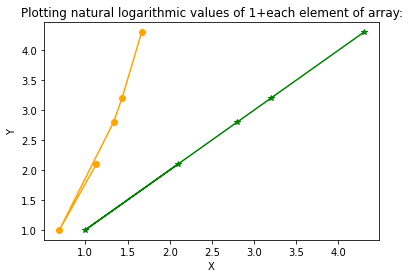# Np.log1p – Python NumPy log1p() Function

NumPy log1p() Function:

Np.log1p: The natural logarithm of (1 + number) which means log(1+number), is calculated using the log1p() function of the NumPy module.

log1p is the inverse of exp(x) – 1.

Syntax:

numpy.log1p(a, out=None)

Parameters

a: This is required. It is an array or an object given as input.

out: This is optional. It is the location where the result will be stored. It must have a shape that the inputs broadcast to if it is provided. If None or not given, a newly allocated array is returned.

Return Value:

The natural logarithm of 1+each element of is returned by the log1p() function of the NumPy module.

## NumPy log1p() Function in Python

Example1

Approach:

• Import numpy module using the import keyword
• Pass some random list as an argument to the array() function of the numpy module to create an array.
• Store it in a variable.
• Print the above-given array.
• Pass the above-given array as an argument to the log1p() function of the numpy module to get the natural logarithmic values of 1+each element of the given array.
• Store it in another variable.
• Print the natural logarithmic values 1+each element of the given array
• The Exit of the Program.

Below is the implementation:

# Import numpy module using the import keyword
import numpy as np
# Pass some random list as an argument to the
# array() function to create an array.
# Store it in a variable.
gvn_arry = np.array([5, 1, 2.4, 10.5, 4])
# Print the above given array.
print("The above given array is:")
print(gvn_arry)
print()
# Pass the above given array as an argument to the log1p() function of the
# numpy module to get the natural logarithmic values of 1+ each element of
# the given array
# Store it in another variable.
rslt = np.log1p(gvn_arry)
# Print the natural logarithmic values of 1+each element of the given array
print("The natural logarithmic values of 1+each element of the given array:")
print(rslt)

Output:

The above given array is:
[ 5. 1. 2.4 10.5 4. ]

The natural logarithmic values of 1+each element of the given array:
[1.79175947 0.69314718 1.22377543 2.44234704 1.60943791]

Example2

Approach:

• Import numpy module using the import keyword
• Import pyplot from the matplotlib module using the import keyword
• Give some random list as static input and store it in a variable.
• Pass the above-given list as an argument to the log1p() function of the numpy module to get the natural logarithmic values of 1+each element of the given array.
• Store it in another variable.
• Store the above input array in another variable for plotting the input array vs input array.
• Plot the input array versus input array with some random color and marker values using the plot() function of the matplotlib module
• Plot the output array versus input array with some other random color and marker values using the plot function of the matplotlib module
• Give the title of the plot using the title() function of the matplotlib module
• Display the plot using the show() function of the matplotlib module.
• The Exit of the Program.

Below is the implementation:

# Import numpy module using the import keyword
import numpy as np
# Import pyplot from the matplotlib module using the import keyword
import matplotlib.pyplot as plt
# Give some random list as static input and store it in a variable.
gvn_arry = [2.1, 1, 2.8, 3.2, 4.3]
# Pass the above-given list as an argument to the log1p() function of the numpy module to
# get the natural logarithmic values of 1+each element of the given array.
# Store it in another variable.
rslt_arry = np.log1p(gvn_arry)
# Store the above input array in another variable for plotting the input array vs input array.
temp_inputarry = [2.1, 1, 2.8, 3.2, 4.3]
# Plot the input array versus input array with some random color and marker values using
# the plot() function of the matplotlib module
plt.plot(gvn_arry, temp_inputarry, color = 'green', marker = "*")
# Plot the output array versus input array with some other random color
# and marker values using the plot function of the matplotlib module
plt.plot(rslt_arry, temp_inputarry, color = 'orange', marker = "o")
# Give the title of the plot using the title() function of the matplotlib module
plt.title("Plotting natural logarithmic values of 1+each element of array:")
plt.xlabel("X")
plt.ylabel("Y")
# Display the plot using the show() function of the matplotlib module.
plt.show()

Output: